# RD Sharma Solutions for Class 9 Maths Chapter 1 Number System Exercise 1.4

RD Sharma Class 9 Mathematics Chapter 1 Exercise 1.4 Number System is provided here. Students can use this study material as a reference tool for studying as well as practicing questions on irrational numbers. The students can enhance their exam preparations by practicing RD Sharma Solutions Class 9 chapter 1 textbook questions for class 9 and score well.

## Download PDF of RD Sharma Solutions for Class 9 Maths Chapter 1 Number System Exercise 1.4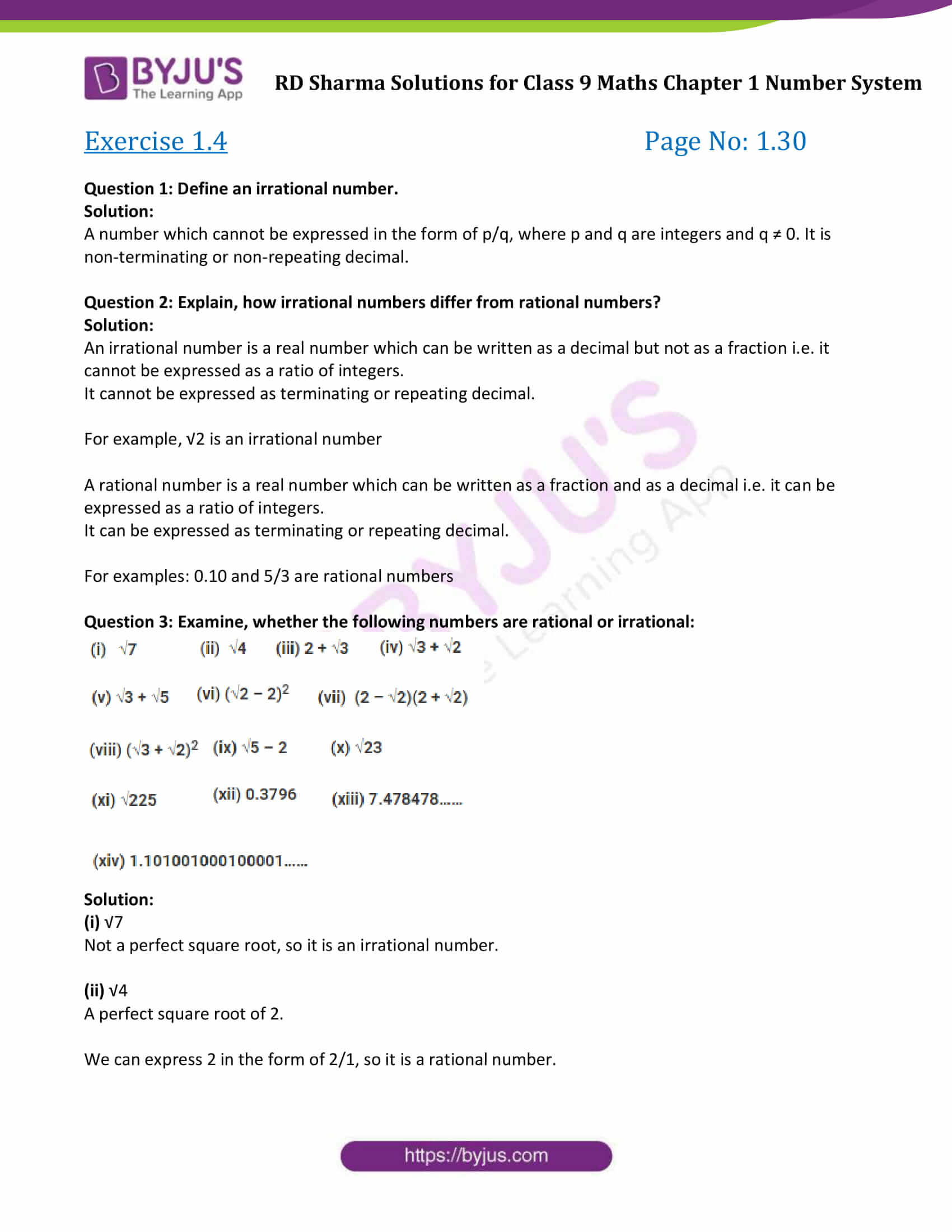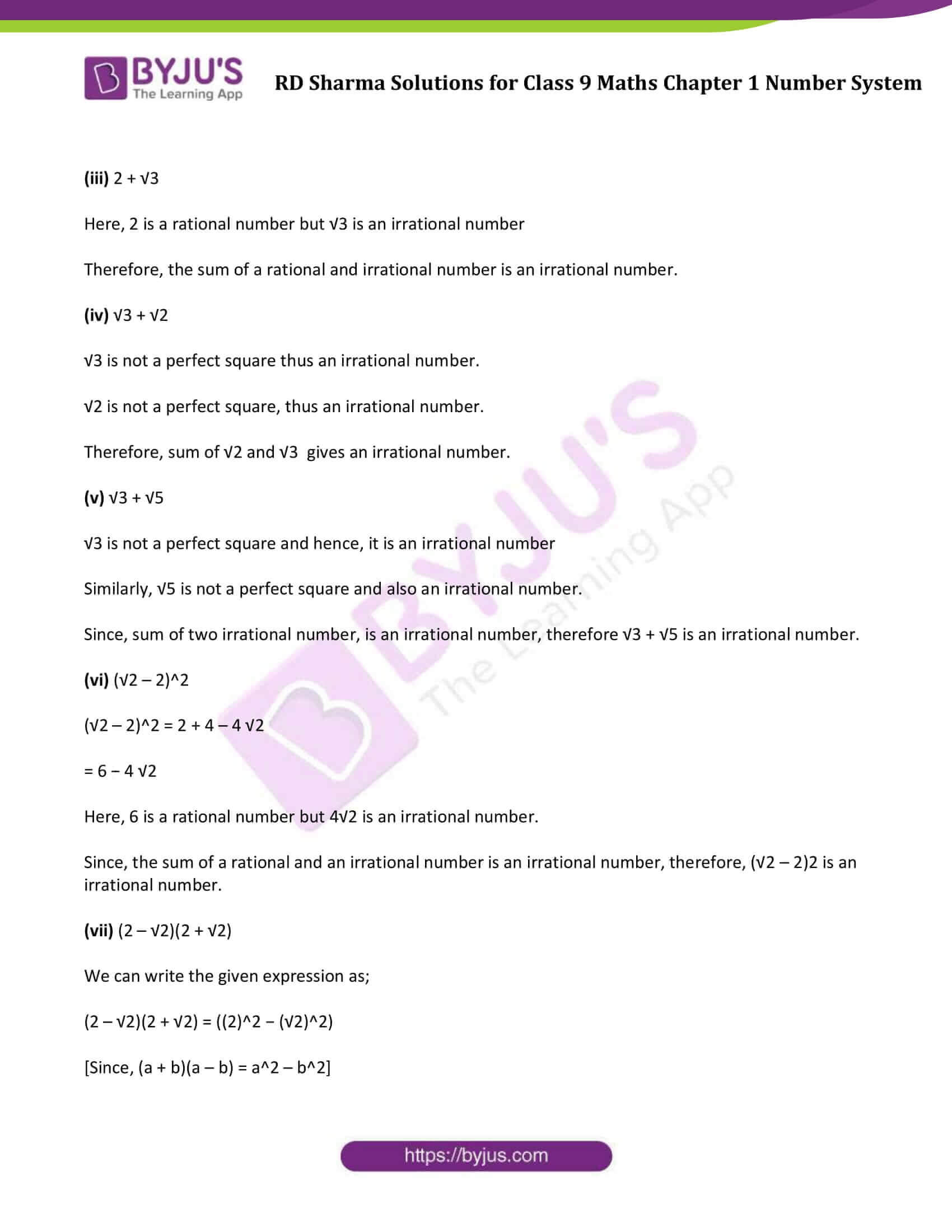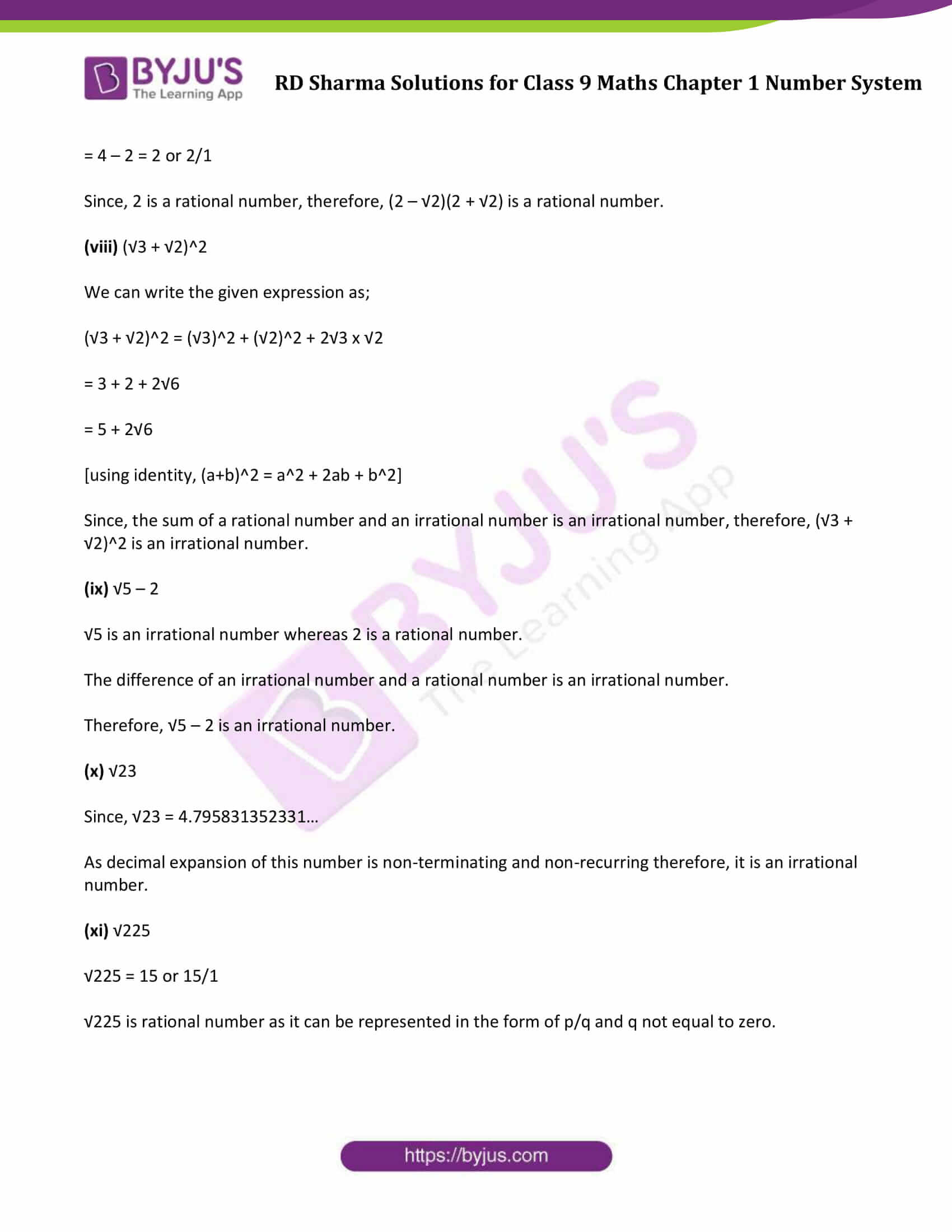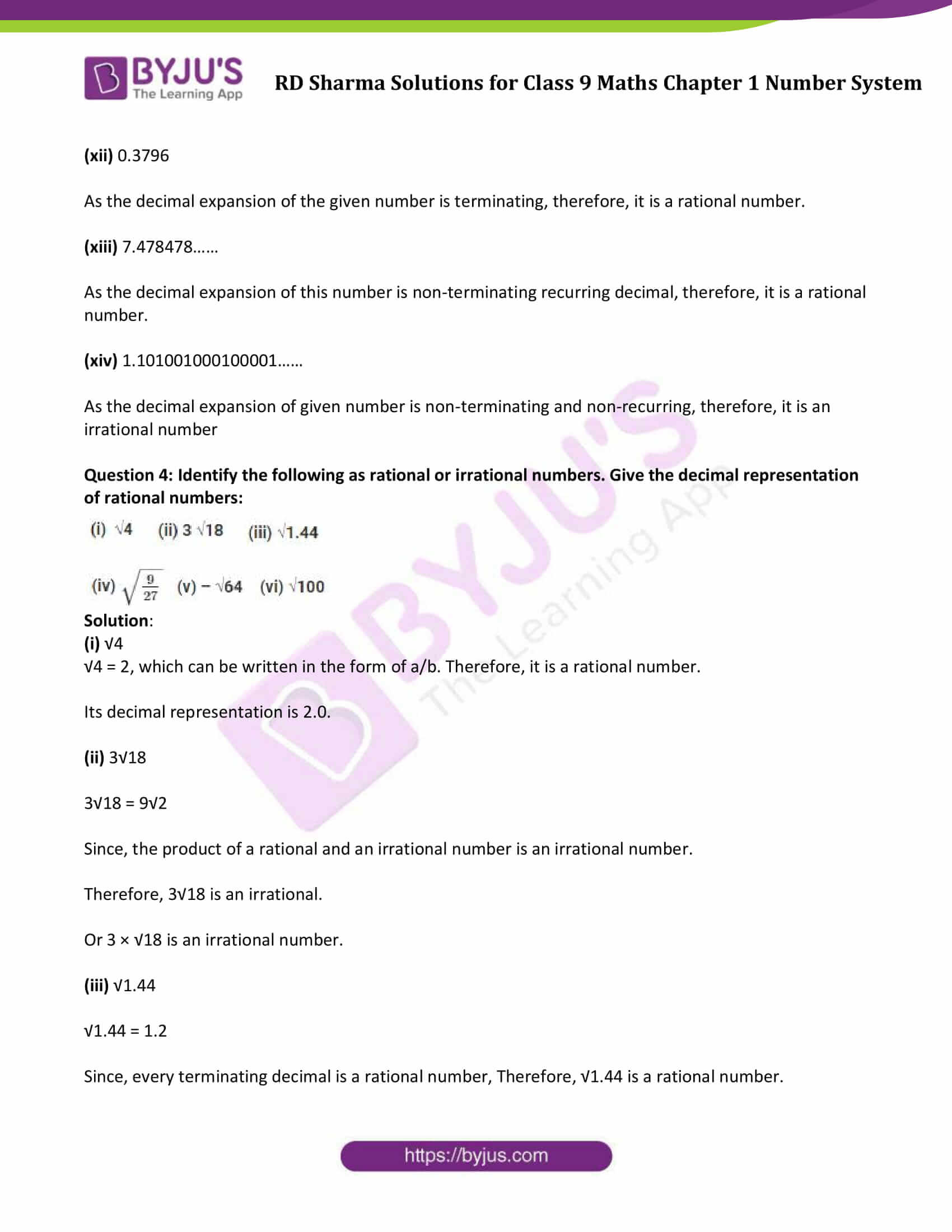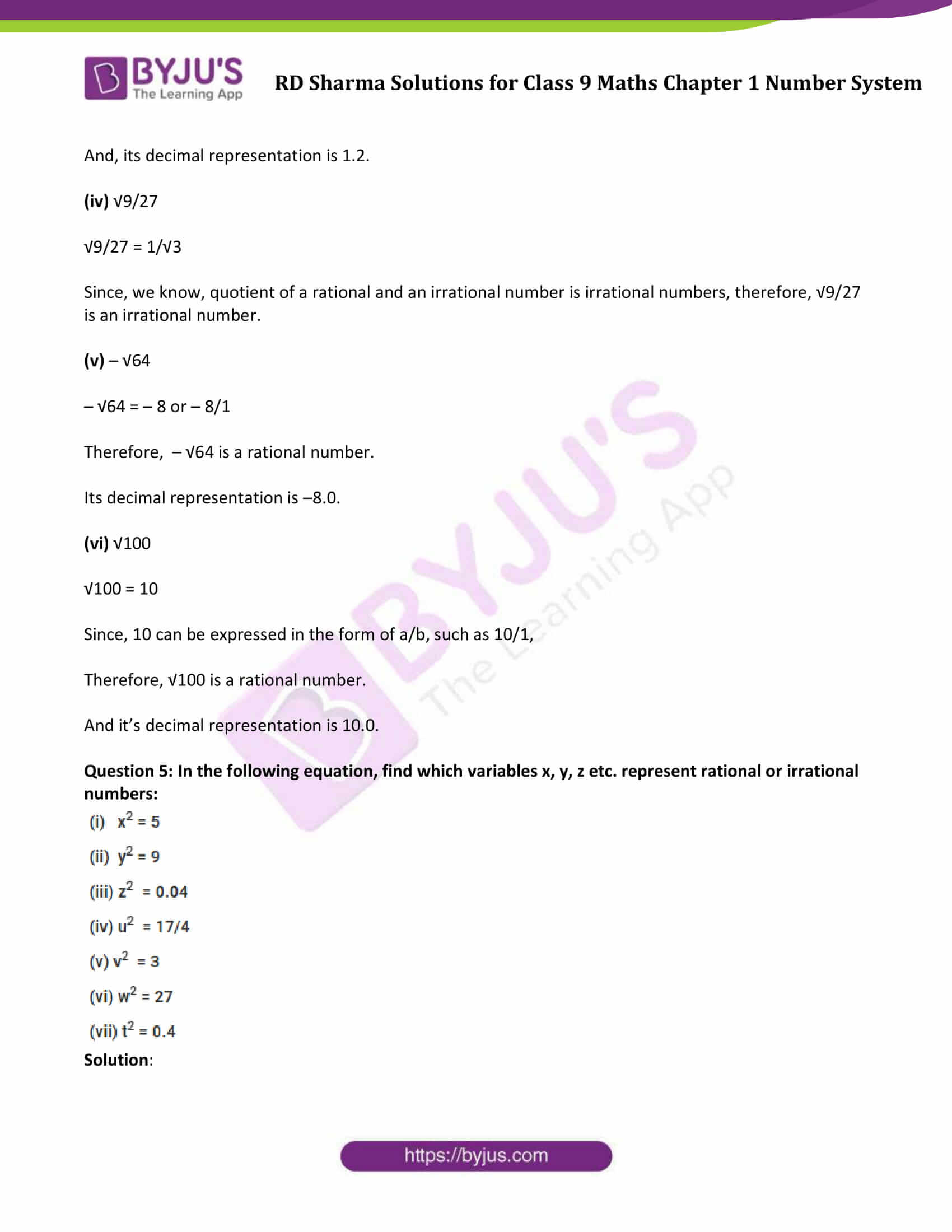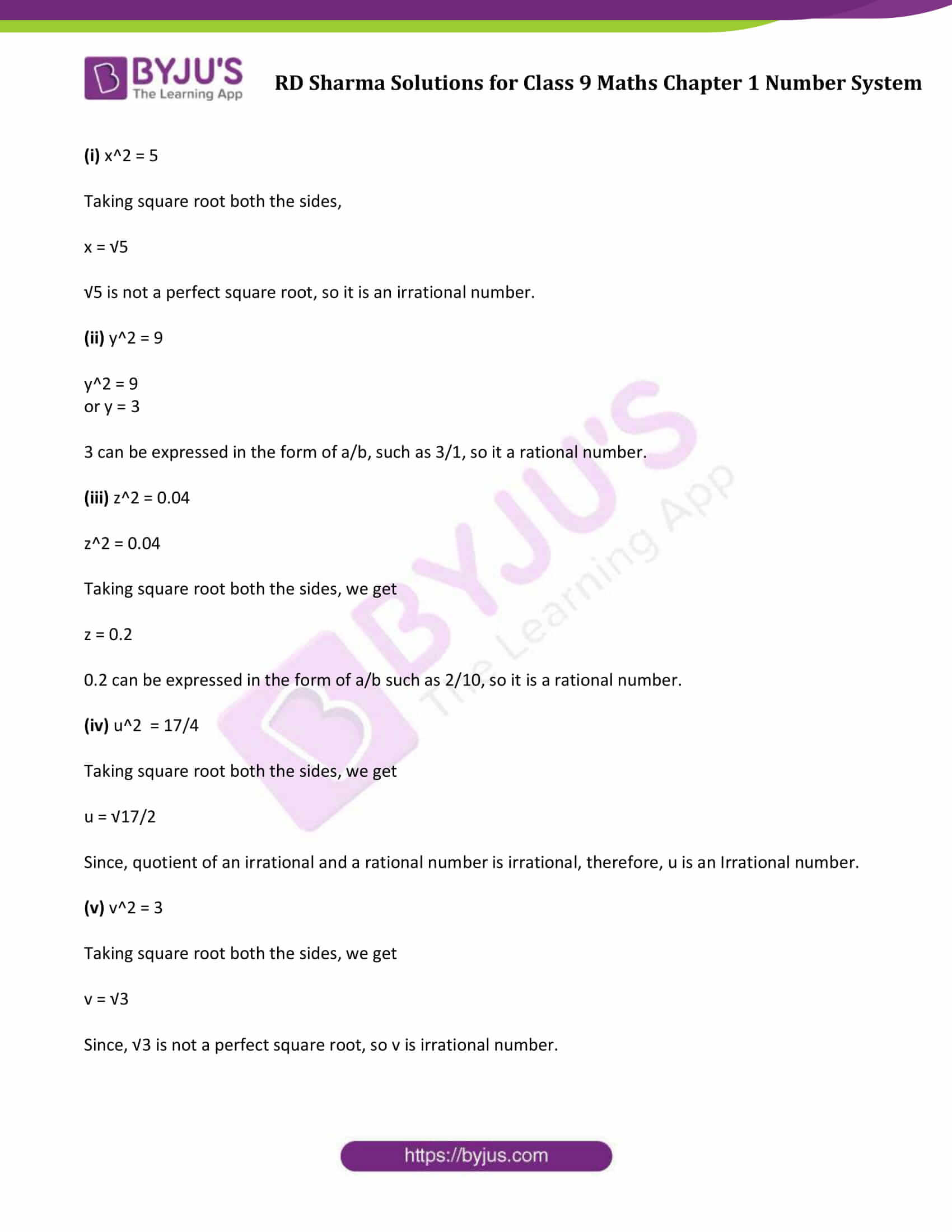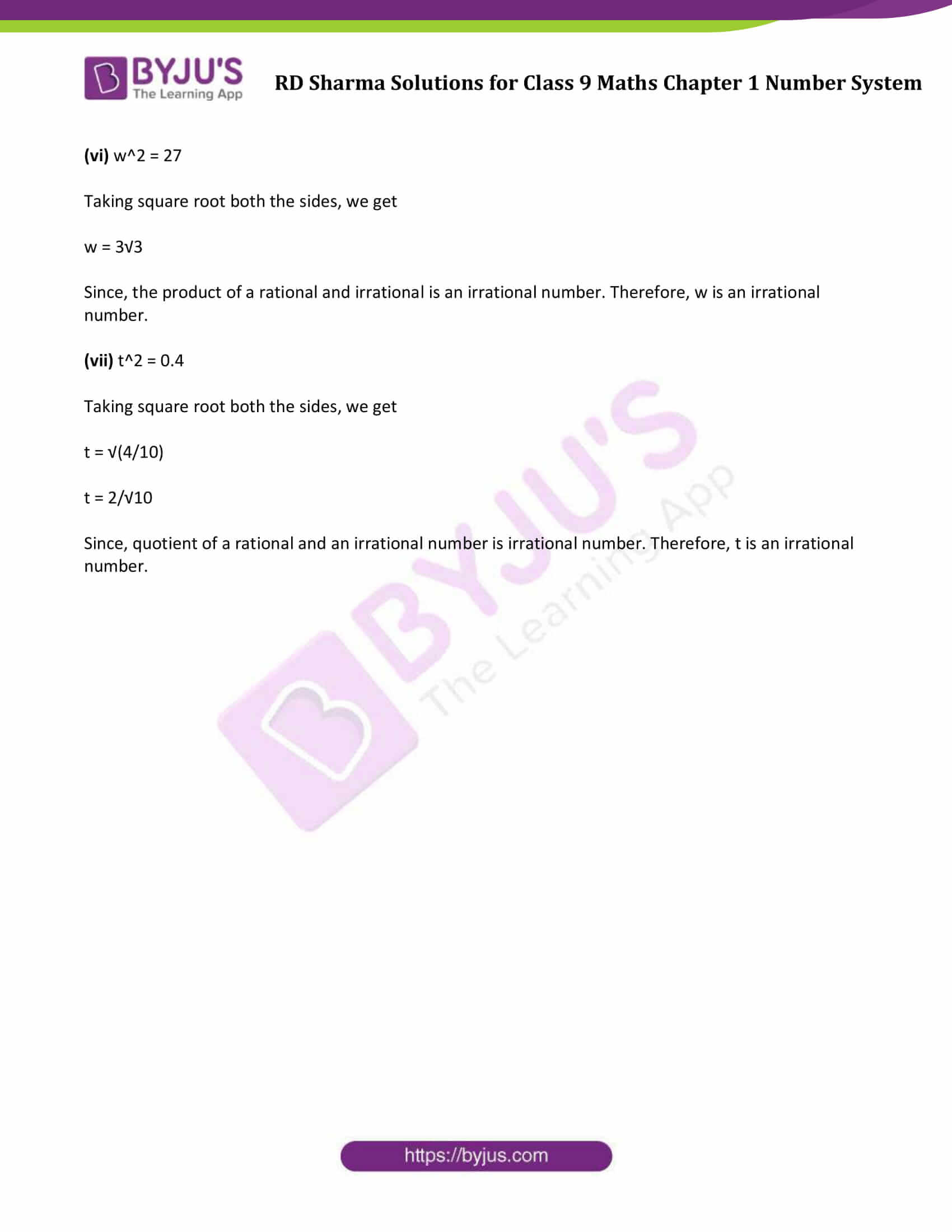## Exercise 1.4

Question 1: Define an irrational number.

Solution:

A number which cannot be expressed in the form of p/q, where p and q are integers and q ≠ 0. It is non-terminating or non-repeating decimal.

Question 2: Explain, how irrational numbers differ from rational numbers?

Solution:

An irrational number is a real number which can be written as a decimal but not as a fraction i.e. it cannot be expressed as a ratio of integers.

It cannot be expressed as terminating or repeating decimal.

For example, √2 is an irrational number

A rational number is a real number which can be written as a fraction and as a decimal i.e. it can be expressed as a ratio of integers.

It can be expressed as terminating or repeating decimal.

For examples: 0.10 and 5/3 are rational numbers

Question 3: Examine, whether the following numbers are rational or irrational: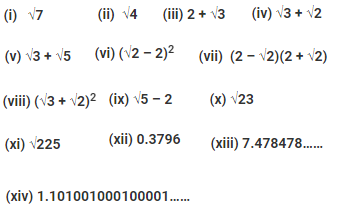Solution:

(i) √7

Not a perfect square root, so it is an irrational number.

(ii) √4

A perfect square root of 2.

We can express 2 in the form of 2/1, so it is a rational number.

(iii) 2 + √3

Here, 2 is a rational number but √3 is an irrational number

Therefore, the sum of a rational and irrational number is an irrational number.

(iv) √3 + √2

√3 is not a perfect square thus an irrational number.

√2 is not a perfect square, thus an irrational number.

Therefore, sum of √2 and √3 gives an irrational number.

(v) √3 + √5

√3 is not a perfect square and hence, it is an irrational number

Similarly, √5 is not a perfect square and also an irrational number.

Since, sum of two irrational number, is an irrational number, therefore √3 + √5 is an irrational number.

(vi) (√2 – 2)2

(√2 – 2)2 = 2 + 4 – 4 √2

= 6 – 4 √2

Here, 6 is a rational number but 4√2 is an irrational number.

Since, the sum of a rational and an irrational number is an irrational number, therefore, (√2 – 2)2 is an irrational number.

(vii) (2 – √2)(2 + √2)

We can write the given expression as;

(2 – √2)(2 + √2) = ((2)2 − (√2)2)

[Since, (a + b)(a – b) = a2 – b2]

= 4 – 2 = 2 or 2/1

Since, 2 is a rational number, therefore, (2 – √2)(2 + √2) is a rational number.

(viii) (√3 + √2)2

We can write the given expression as;

(√3 + √2)2 = (√3)2 + (√2)2 + 2√3 x √2

= 3 + 2 + 2√6

= 5 + 2√6

[using identity, (a+b)2 = a2 + 2ab + b2]

Since, the sum of a rational number and an irrational number is an irrational number, therefore, (√3 + √2)2 is an irrational number.

(ix) √5 – 2

√5 is an irrational number whereas 2 is a rational number.

The difference of an irrational number and a rational number is an irrational number.

Therefore, √5 – 2 is an irrational number.

(x) √23

Since, √23 = 4.795831352331…

As decimal expansion of this number is non-terminating and non-recurring therefore, it is an irrational number.

(xi) √225

√225 = 15 or 15/1

√225 is rational number as it can be represented in the form of p/q and q not equal to zero.

(xii) 0.3796

As the decimal expansion of the given number is terminating, therefore, it is a rational number.

(xiii) 7.478478……

As the decimal expansion of this number is non-terminating recurring decimal, therefore, it is a rational number.

(xiv) 1.101001000100001……

As the decimal expansion of given number is non-terminating and non-recurring, therefore, it is an irrational number

Question 4: Identify the following as rational or irrational numbers. Give the decimal representation of rational numbers: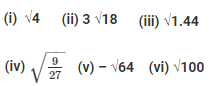Solution:

(i) √4

√4 = 2, which can be written in the form of a/b. Therefore, it is a rational number.

Its decimal representation is 2.0.

(ii) 3√18

3√18 = 9√2

Since, the product of a rational and an irrational number is an irrational number.

Therefore, 3√18 is an irrational.

Or 3 × √18 is an irrational number.

(iii) √1.44

√1.44 = 1.2

Since, every terminating decimal is a rational number, Therefore, √1.44 is a rational number.

And, its decimal representation is 1.2.

(iv) √9/27

√9/27 = 1/√3

Since, we know, quotient of a rational and an irrational number is irrational numbers, therefore, √9/27 is an irrational number.

(v) – √64

– √64 = – 8 or – 8/1

Therefore, – √64 is a rational number.

Its decimal representation is –8.0.

(vi) √100

√100 = 10

Since, 10 can be expressed in the form of a/b, such as 10/1,

Therefore, √100 is a rational number.

And it’s decimal representation is 10.0.

Question 5: In the following equation, find which variables x, y, z etc. represent rational or irrational numbers: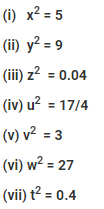Solution:

(i) x2 = 5

Taking square root both the sides,

x = √5

√5 is not a perfect square root, so it is an irrational number.

(ii) y2 = 9

y2 = 9

or y = 3

3 can be expressed in the form of a/b, such as 3/1, so it a rational number.

(iii) z2 = 0.04

z2 = 0.04

Taking square root both the sides, we get

z = 0.2

0.2 can be expressed in the form of a/b such as 2/10, so it is a rational number.

(iv) u2 = 17/4

Taking square root both the sides, we get

u = √17/2

Since, quotient of an irrational and a rational number is irrational, therefore, u is an Irrational number.

(v) v2 = 3

Taking square root both the sides, we get

v = √3

Since, √3 is not a perfect square root, so v is irrational number.

(vi) w2 = 27

Taking square root both the sides, we get

w = 3√3

Since, the product of a rational and irrational is an irrational number. Therefore, w is an irrational number.

(vii) t2 = 0.4

Taking square root both the sides, we get

t = √(4/10)

t = 2/√10

Since, quotient of a rational and an irrational number is irrational number. Therefore, t is an irrational number.

## RD Sharma Solutions for Class 9 Maths Chapter 1 Number System Exercise 1.4

RD Sharma Solutions Class 9 Maths Chapter 1 Number System Exercise 1.4 is based on following topics and subtopics:

• Irrational Numbers.
• A number is an irrational number if it has a non-terminating and non-repeating decimal representation.
• Some useful results on Irrational Numbers.